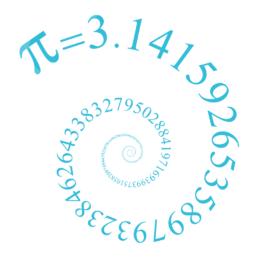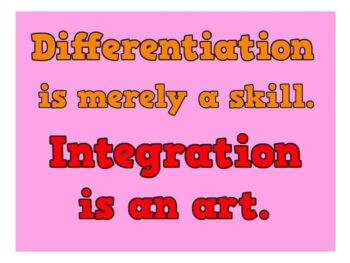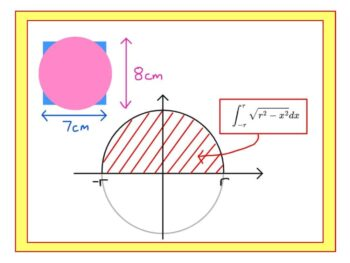Bournemouth Maths Tutor Barney M-TQuote of the week: DIFFERENTIATION IS A SKILL. INTEGRATION IS AN ART.

I was delighted to find this quote at the start of Seán M. Stewart‘s book “How to Integrate it“.One of the main differences between GCSE and A-level maths is the introduction of calculus. This branch of maths makes sense of the infinitely small, allowing us to deal with quantities that vary continuously (often over time)WHAT’S THE POINT OF INTEGRATION BY SUBSTITUTION?

A PUZZLE: which of these shapes is larger: a square of side 7cm or a circle or diameter 8cm? Well that’s easy to answer if you know that the area of a circle is $A=\pi r^2$ but how do we know that $A=\pi r^2$ ?? Here is a particularly rigorous way that uses many of